Revisit the Motor+Gearbox+Propeller Model8 min read

# Revisit the Motor+Gearbox+Propeller Model8 min read

It was fun to just mess with the motor-propeller differential equation and linearization in my earlier post. However, when I got into looking-up some rough parameter estimates and performing dimensional analysis (seeing if the units worked-out right for me in the equations) I ran into some problems.

I realized I was not only needing numbers, but I wasn’t comfortable with the units either! This means no controller design until I get a better understanding of the, “plant” (the thing we want to control: the propeller speed via the motor and gearbox if any).

The picture for this post shows a Mr. Fred Ernest Weick (1899-1993). I show him because I learned of him as I dug into some details relating to this post. I got stuck trying to understand parameters in the motor+gearbox+propeller equation in my earlier post as I was moving ahead to a speed controller for each propeller.

Thanks to the internet I was able to find additional resources. Eventually I encountered a book by Mr. Wieck, and learned he was quite a force in the world of aviation. I found a PDF online of his 1926 book shown here. Mr. Weick did a lot of work on propellers and designed some aircraft. Safe civilian aircraft design appears to have been one of his motivations. I didn’t so much study his materials as get interested in his history. It is enjoyable to learn about aviation history.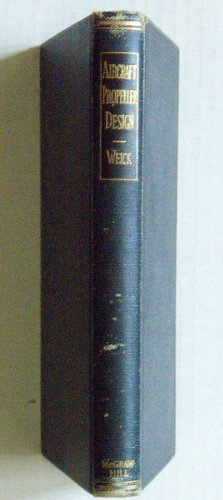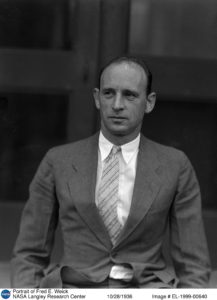## Returning to our Motor+Gearbox+Propeller Equation

I derived this equation earlier to match what Bouabdallah presents in his paper (see his equation 14). I went step-by step to illustrate his result.Where…Trouble is, I had no idea where a couple of the terms originated. It was fine to work the equation earlier, but I did not fully understand the electro-mechanics through the gearbox to the propeller. I was, doing the math but not prepared to solve the problem!

I reworked the derivation many, many times after looking at more references. The biggest hang-up for me was theterm in the equations. ‘r’ is the gearbox ratio. I could not figure out how it came to be cubed in these equations!

I kept re-reading, re-deriving, and looking for examples in other sources that would make it clear to me how this simple gearbox ratio gets cubed. In the end, it was very simple but it took me some hours off-and-on to finally, “get it”. I’ll share the process because this is one of my goals with these writings: share all the hang-ups and as many details as I can fill-in to illustrate not only the solutions to problems, but my struggles to understand all manner of details from the simple to the complicated. Sometimes they will be comical!

### My, “sticking-point”

I was stumped by a small section of my primary reference paper for this project. In my earlier post I was happy to go through the linearization and get to the same, “answer” for the A, B, and C terms but I didn’t really understand one part of it: the propeller load on the motor through the gearbox.

Below is what I had to go on: steps 11-14 (on the left) in the paper together with the term descriptions to the right. The one step that reads, “By introducing the propeller and the gearbox models…” is what challenged my understanding. This is wherein equation 12 becomesin equation 13. I did not understand how this leap was made.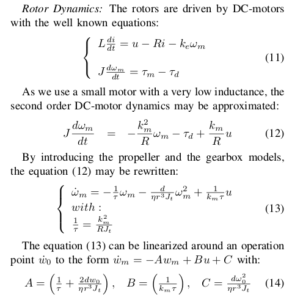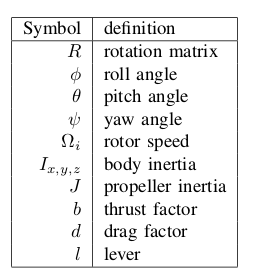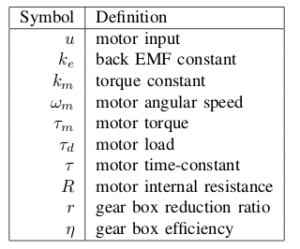### My understanding of the propeller load terms…

• I was OK with the () part that told me that the propeller put a load on the motor proportional to the square of the prop rotational speed.
• This is consistent with the last post where the NASA equations had squared airspeed difference for total force out of the prop. It makes sense that we have a squared rotational term here for torque.
• I had no idea where to find some example values for, ‘d’
• I was not altogether confident I understood the context but I took it to imply the drag of the air resisting the rotation of a propeller.
• How did the moment of inertia go fromto?
• I know when using motors with shafts, gears, and, “loads” we want to, “refer” all of the downstream complications to the motor shaft in one, “lumped” parameter so that our problem looks like a motor spinning a disk.
• We, “lump” all the complications into an equivalent moment of inertia• I figured this is whatrepresents but was not sure how to arrive at it through the gearbox
• Lumped inertia term aside, my main hang-up was the cubed gear-ratio in the denominator.
• Typically we apply gear ratios as a fractional step-up in torque and down in rotational speed or vice-versa.
• To see the gear ratio cubed aswas a complete blind spot for me.

### The Eye-opener

Reading another paper it suddenly clicked in my mind. It’s pretty simple. Most every time I am stuck I realize the resolution was simple later. This is why I don’t mind being stuck. I know if I just stay at a problem typically my mental block will clear.

Here the paper usesinstead of, ‘r’ so I’ll stick with the paper’s notation for a bit.

I knew these simple gearbox relationships but I had not systematically applied them to-and-from the motor to the propeller for the load model:tells us that the torque the motor sees is equal to the load torque at the propeller divided by the gear ratio (from the above gearbox equation).

This is the, “motor load”term in equation (12) from our paper above, so here we see one gear ratio term in the denominator.

Now, on the propeller-side of the gearbox we have a simple relationship for torque as a function of propeller speed squared:BUT we also know thatSo it all simplifies to…There we have it! Theterm is ourterm! When we work this back through the equations we’ll get the A, B, and C terms the same as the Bouabdallah paper.

Here’s the paper that got me thinking correctly. This is an example of one method I use when I am stuck: just look at more-and-more source material until somebody’s treatment of a subject offers a different twist, or maybe includes an additional step, or diagram, or anything that helps you see it clearly. In the end, you can see I am combining the source materials. The Bouabdallah paper is still a primary reference, but this one and others are good support material. They help deepen understanding of each step in the process.

lab2_rotary_dynamics

### Total moment of inertia as seen at the motor shaft

Let’s focus on line (3) in the PDF above so we can determine what our moment of inertia will be, referred to the motor shaft.is simply replacing thepropeller-side torque with thedefinition of torque (the F=ma for rotations about an axis).after we use the gearbox relationships above and replace the load-sideacceleration term with the motor-side equivalent through the gearbox.

That fractional term is the propeller load inertia as the motor, “sees” it on the motor side of the gearbox. We’ve referred it directly to the motor shaft. The total inertial load on the motor is then just the sum of the motor’s and this load inertia mapped back to the motor shaft.is then the inertia of the motor and the load referred to the motor shaft. In the case of the load, it is mapped-back to the motor shaft through the gearbox via the denominator term.

### End Result of this exercise

My confusion over theterm turned-out to be a blessing. I spent more time (re)working the electro-mechanical motor equations than I would have done. I accidentally became much more confident in all the parameters and my working knowledge of the derivation of the final differential equation and it’s terms:I really had TWO problems to overcome:

1. My problem with the cubed gear ratio
2. The total rotational inertia seen by the motor.

In my focus on settling #1 in my mind, I bumped into #2, and as indicated above I now have the equation I need to compute### The, ‘d’ term in the propeller load equation

The trick with the, ‘d’ term is it needs to multiply anterm to produce a torque. If I had studied any aeronautics I probably would have had a reference point for this, but I didn’t.

I eventually found a term in, “Modelling if the ETH Helicopter Laboratory Process” by Magnus Gafvert that appears to employ comparable motors and propellers for a small helicopter test stand. Here I found the following parameter listed:

“Aerodynamic torque for rotor R:(page 9, Gafvert) for what looks like a comparable hobby-sized propeller lab set-up. I can at least get started with this order-of-magnitude estimate. Those units will give me a torque (Nm) when I multiply by(nominal propeller speed around linearization point).

Gafvert references Mr. Weick in his paper, which is what sent me marveling at some engineering history. I could start my Aerodynamic studies with his 90 year-old book!

## Conclusion

This post has been a great exercise in overcoming confusion. In my experience, grinding-through my own mental blocks and retreating from the blind alley is a big part of problem solving. Maybe that’s why we call them, “problems” and not immediately, “solutions”! I also come away with a much deeper understanding of the DC motor equations from my youthful days in school. I cemented good knowledge in preparing this report.